19 January 2018

# Creating a building block using polygons

### How to create a building block using polygons and polygon generating function from geometries module

(For more detailed information on making cells see also Make and put a Cell)

We create a building block from a list of points (x, y) in a custom mask layer. The building  block is defined as a cell via the ‘with’ statement. Inside the cell we create a Polygon object from the points which is put in the cell. To be able to connect to our new building block we need to define pins via Pin objects, which we also put in the cell. Note that according to the Nazca convention (for chain connections) pins point outwards from a building block. To show the pins in the GDS we call put_stub.

```# example created by Bright Photonics

import nazca as nd

# create a layer and define its accuracy

# create a building block from Polygon points
with nd.Cell('building_block') as bb:

bb_body = [(5.0, -5.0), (5.0, -1.0), (0.0, -1.0), (0.0, 1.0),
(5.0, 1.0), (5.0, 5.0), (35.0, 5.0), (35.0, 3.5),
(40.0, 3.5), (40.0, 1.5), (35.0, 1.5), (35.0, -1.5),
(40.0, -1.5), (40.0, -3.5), (35.0, -3.5), (35.0, -5.0)]
nd.Polygon(points=bb_body, layer='layer3').put(0)

nd.Pin('a0').put(0, 0, 180)
nd.Pin('b0').put(40, 2.5, 0)
nd.Pin('b1').put(40, -2.5, 0)
nd.put_stub()

bb.put(0)
bb.put('b1')

nd.export_gds()```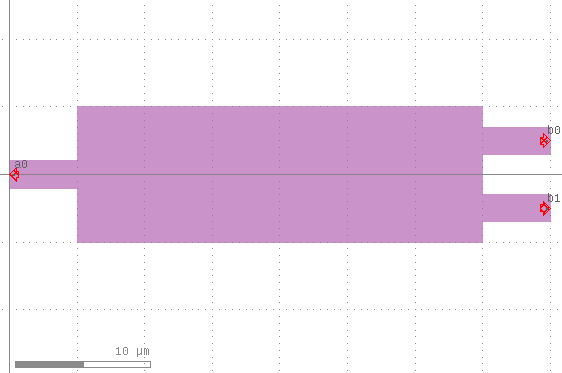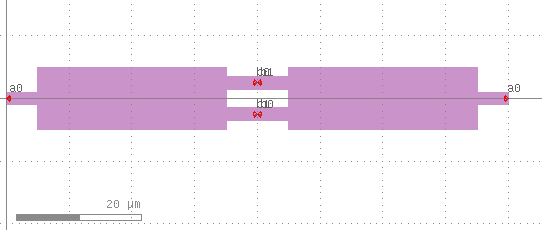You can also use predefined polygon generating functions from the Nazca geometries module.

```# example created by Bright Photonics

import nazca as nd
import nazca.geometries as geom

# create a layer and define its accuracy

# create a building block from predefined geometries
with nd.Cell('building_block') as bb:

bb_io = geom.box(length=5, width=2)
bb_body = geom.box(length=30, width=10)

nd.Polygon(points=bb_io, layer='layer3').put(0)
nd.Polygon(points=bb_body, layer='layer3').put(5)
nd.Polygon(points=bb_io, layer='layer3').put(35, 2.5)
nd.Polygon(points=bb_io, layer='layer3').put(35, -2.5)

nd.Pin('a0').put(0, 0, 180)
nd.Pin('b0').put(40, 2.5, 0)
nd.Pin('b1').put(40, -2.5, 0)
nd.put_stub()

bb.put(0)
bb.put('b1')

nd.export_gds()```A bounding box can be put around the cell to better visualize and/or extend connection options of the building block.

```# example created by Bright Photonics

import nazca as nd
import nazca.geometries as geom

# create a layer and define its accuracy

# create a building block from predefined geometries
with nd.Cell('building_block') as bb:

bb_io = geom.box(length=5, width=2)
bb_body = geom.box(length=30, width=10)

nd.Polygon(points=bb_io, layer='layer3').put(0)
nd.Polygon(points=bb_body, layer='layer3').put(5)
nd.Polygon(points=bb_io, layer='layer3').put(35, 2.5)
nd.Polygon(points=bb_io, layer='layer3').put(35, -2.5)

nd.Pin('a0').put(0, 0, 180)
nd.Pin('b0').put(40, 2.5, 0)
nd.Pin('b1').put(40, -2.5, 0)
nd.put_stub()

# Put boundary around the building block
nd.put_boundingbox('org', length=40, width=10)

bb.put(0)
bb.put('b1')

nd.export_gds()```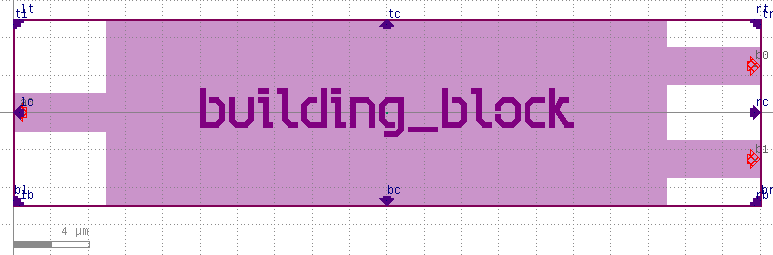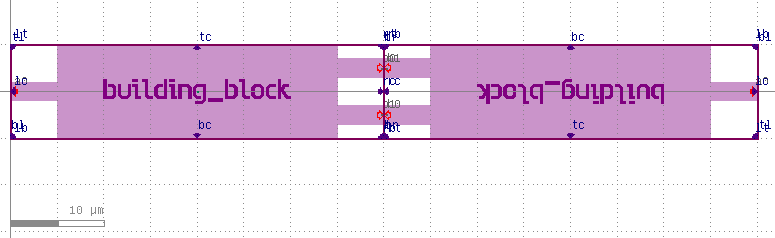## Related Tutorials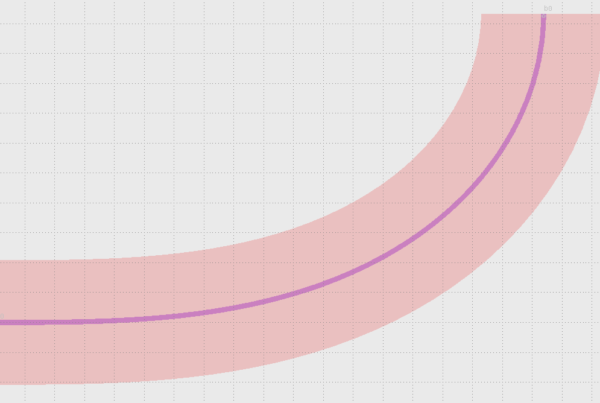In this example we show how to use Euler bends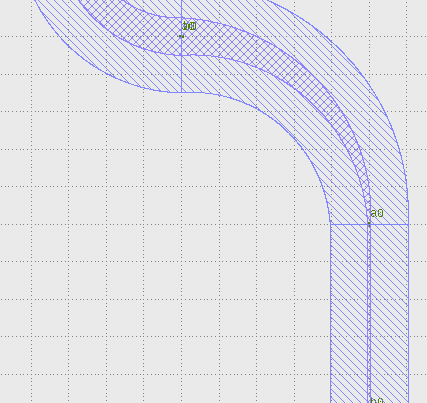In this example we show how to create a free form parametric curve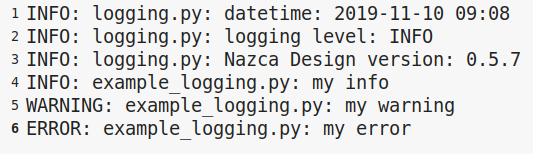In this example we show how to log your layout.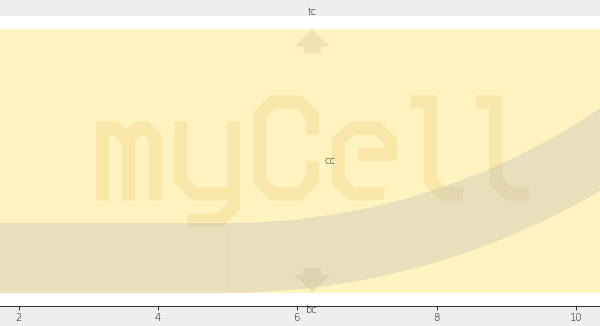In this example we show how to create xsections and layers